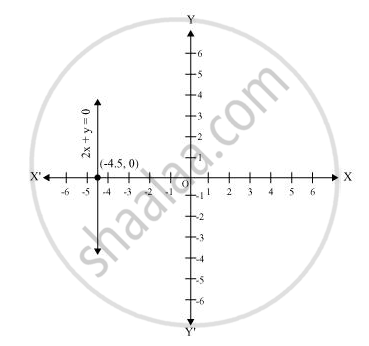Share

# Give the geometric representations of 2x + 9 = 0 as an equation in two variables. - CBSE Class 9 - Mathematics

ConceptEquations of Lines Parallel to the X-axis and Y-axis

#### Question

Give the geometric representations of 2x + 9 = 0 as an equation in two variables.

#### Solution

In two variables, 2x + 9 = 0 represents a straight line passing through point (−4.5, 0) and parallel to y-axis. It is a collection of all points of the plane, having their x-coordinate as 4.5.Is there an error in this question or solution?

#### APPEARS IN

NCERT Solution for Mathematics Class 9 (2018 to Current)
Chapter 4: Linear Equations in two Variables
Ex. 4.40 | Q: 2.2 | Page no. 77

#### Video TutorialsVIEW ALL 

Solution Give the geometric representations of 2x + 9 = 0 as an equation in two variables. Concept: Equations of Lines Parallel to the X-axis and Y-axis.
S